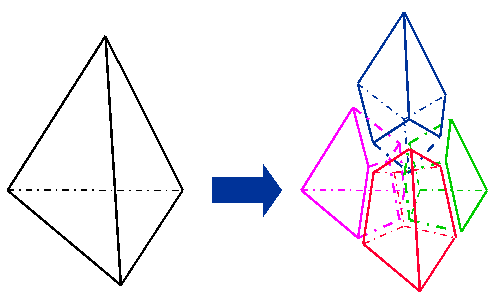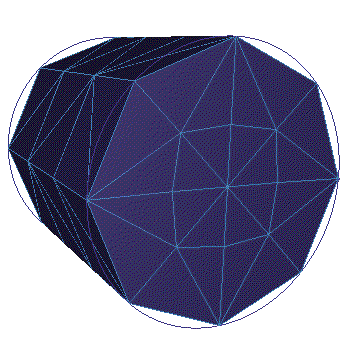# THex

Applies to: Volumes

Summary: Converts a tetrahedral mesh into a hexahedral mesh.

Syntax:

THex Volume <range>

Discussion:

The THex command splits each tetrahedral element in a volume into four hexahedral elements, as shown in Figure 1. This is done by splitting each edge and face at its midpoint, and then forming connections to the center of the tet.

When THexing merged volumes, all of the volumes must be THexed at the same time, in a single command. Otherwise, meshes on shared surfaces will be invalid. An example of the THex algorithm is shown in Figure 2.Figure 1. Conversion of a tetrahedron to four hexahedra, as performed by the THex algorithm.

.Figure 2. A cylinder before and after the THex algorithm is applied.